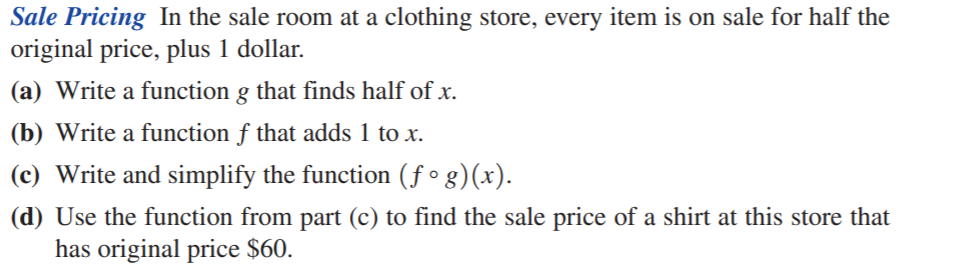### Still have math questions?

Algebra
QuestionSale Pricing In the sale room at a clothing store, every item is on sale for half the original price, plus $$1$$ dollar.

(a) Write a function $$g$$ that finds half of $$x$$ .

(b) Write a function $$f$$ that adds $$1$$ to $$x$$ .

(c) Write and simplify the function $$( f \circ g ) ( x )$$ .

(d) Use the function from part (c) to find the sale price of a shirt at this store that has original price $$\ 60$$ .

$$\left. \begin{array} { l } { \text { (d) } \ 9600 \text { 111. (a) } g ( x ) = \frac { 1 } { 2 } x \quad \text { (b) } f ( x ) = x + 1 } \\ { \text { (c) } ( f \circ g ) ( x ) = f ( g ( x ) ) = g ( x ) + 1 = \frac { 1 } { 2 } x + 1 } \\ { \text { (d) } ( f \circ g ) ( 60 ) = \frac { 1 } { 2 } ( 60 ) + 1 = 31 \text { (dollars) } } \end{array} \right.$$